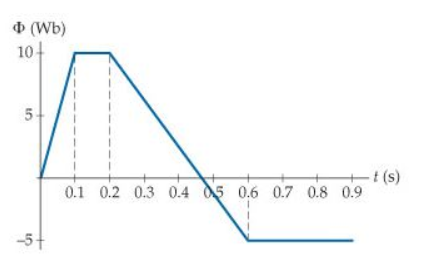# Problem: The figure (Figure 1) shows the magnetic flux through a single-loop coil as a function of time.Part AWhat is the induced emf in the coil at t=0.050s?Part BWhat is the induced emf in the coil at t=0.15s?Part CWhat is the induced emf in the coil at t=0.50s?

###### FREE Expert Solution

Induced emf:

$\overline{){\mathbf{\epsilon }}{\mathbf{=}}{\mathbf{-}}\frac{\mathbf{d}\mathbf{\varphi }}{\mathbf{d}\mathbf{t}}}$'

This implies that the induced emf is the negative of the slope of the flux versus time graph.

$\overline{){\mathbf{\epsilon }}{\mathbf{=}}{\mathbf{-}}{\mathbf{\left(}}\frac{{\mathbf{\varphi }}_{\mathbf{2}}\mathbf{-}{\mathbf{\varphi }}_{\mathbf{1}}}{{\mathbf{t}}_{\mathbf{2}}\mathbf{-}{\mathbf{t}}_{\mathbf{1}}}{\mathbf{\right)}}}$

Part A

At t = 0.05 s, the slope is:

${\mathbf{\epsilon }}{\mathbf{=}}{\mathbf{-}}{\mathbf{\left(}}\frac{\mathbf{10}\mathbf{-}\mathbf{0}}{\mathbf{0}\mathbf{.}\mathbf{1}\mathbf{-}\mathbf{0}}{\mathbf{\right)}}$

91% (73 ratings)###### Problem Details

The figure (Figure 1) shows the magnetic flux through a single-loop coil as a function of time.Part A
What is the induced emf in the coil at t=0.050s?

Part B
What is the induced emf in the coil at t=0.15s?

Part C
What is the induced emf in the coil at t=0.50s?